# Solved 2019 Question Paper ICSE Class 10 Physics

## Section I (40 Marks)

#### Question 1(a)

The diagram below shows a claw hammer used to remove a nail: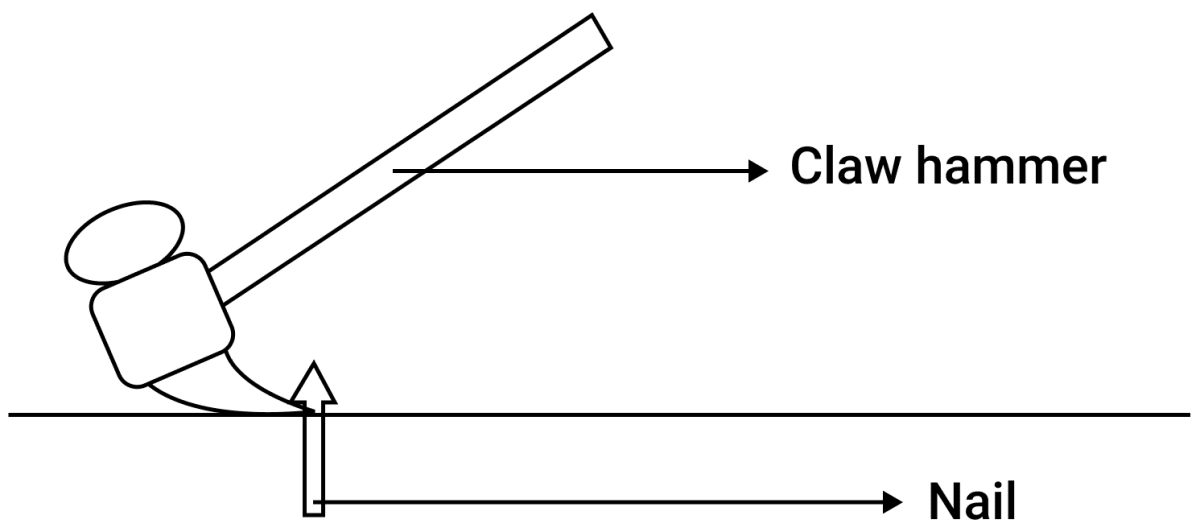(i) To which class of lever does it belong ?

(ii) Give one more example of the same class of lever mentioned by you in (i) for which the mechanical advantage is greater than one.

(i) A claw hammer belongs to class I lever.

(ii) Another example of class I lever is shears (used to cut metal sheets)

#### Question 1(b)

Two bodies A and B have masses in the ratio 5:1 and their kinetic energies are in the ratio 125:9. Find the ratio of their velocities. 

Given,

$\dfrac{m_A}{m_B} = \dfrac{5}{1} \\[0.5em] \dfrac{K_A}{K_B} = \dfrac{125}{9}$

Since,

$\dfrac{K_A}{K_B} = \dfrac{\dfrac{1}{2}m_Av_A^2}{\dfrac{1}{2}m_Bv_B^2} \\[0.5em] = \Big(\dfrac{m_A}{m_B}\Big) \times \Big(\dfrac{v_A^2}{v_B^2}\Big)$

Substituting the values we get,

$\dfrac{125}{9} = \dfrac{5}{1} \times \dfrac{v_A^2}{v_B^2} \\[0.5em] \Rightarrow \dfrac{v_A^2}{v_B^2} = \dfrac{125 \times 1}{9 \times 5} \\[0.5em] \Rightarrow \dfrac{v_A}{v_B} = \sqrt{\dfrac{25}{9}} = \dfrac{5}{3} \\[0.5em]$

Hence, ratio between velocities of A and B is 5 : 3

#### Question 1(c)

(i) Name the physical quantity which is measured in calories.

(ii) How is calorie related to the S.I. unit of that quantity? 

(i) Heat energy is measured in calories.

(ii) 1 cal = 4.2 J where joule is the S.I. unit of heat energy.

#### Question 1(d)

(i) Define couple.

(ii) State the S.I. unit of moment of couple. 

(i) Two equal and opposite parallel forces, not acting along the same line, form a couple.

(ii) The S.I. unit of moment of couple is Newton x meter or Nm.

#### Question 1(e)

(i) Define critical angle.

(ii) State one important factor which affects the critical angle of a given medium. 

(i) Critical angle is the angle of incidence in the denser medium corresponding to which the angle of refraction in the rarer medium is 90°.

(ii) The refractive index of the medium affects the critical angle of a given medium.

#### Question 2(a)

An electromagnetic radiation is used for photography in fog.

(ii) Why is this radiation mentioned by you, ideal for this purpose? 

(ii) Infra-Red radiation has larger wavelength hence, it is scattered least among visible radiations and thus have deep penetration even in fog.

#### Question 2(b)

(i) What is the relation between the refractive index of water with respect to air (aμw) and the refractive index of air with respect to water (wμa)

(ii) If the refractive index of water with respect to air (aμw) is $\dfrac{5}{3}$. Calculate the refractive index of air with respect to water (wμa). 

(i) aμw = $\dfrac{1}{_wμ_a}$ i.e., they are inverse of each other.

(ii) Given, aμw = $\dfrac{5}{3}$

Therefore, wμa = $\dfrac{3}{5}$ = 0.6

#### Question 2(c)

The specific heat capacity of a substance A is 3,800 J kg-1K-1 and that of a substance B is 400 J kg-1K-1. Which of the two substances is a good conductor of heat? Give a reason for your answer. 

Substance B is a good conductor of heat energy. For the same heat energy and same mass, the rise in temperature of B will be more hence, B is a good conductor of heat.

#### Question 2(d)

A man playing a flute is able to produce notes of different frequencies. If he closes the holes near his mouth, will the pitch of the note produced, increase or decrease? Give a reason. 

When the holes near the mouth are closed, the effective length of air column increases so the pitch of sound decreases as the frequency of note produced is inversely proportional to the length of air column.

#### Question 2(e)

The diagram below shows a light source P embedded in a rectangular glass block ABCD of critical angle 42°. Complete the path of the ray PQ till it emerges out of the block. [Write necessary angles.]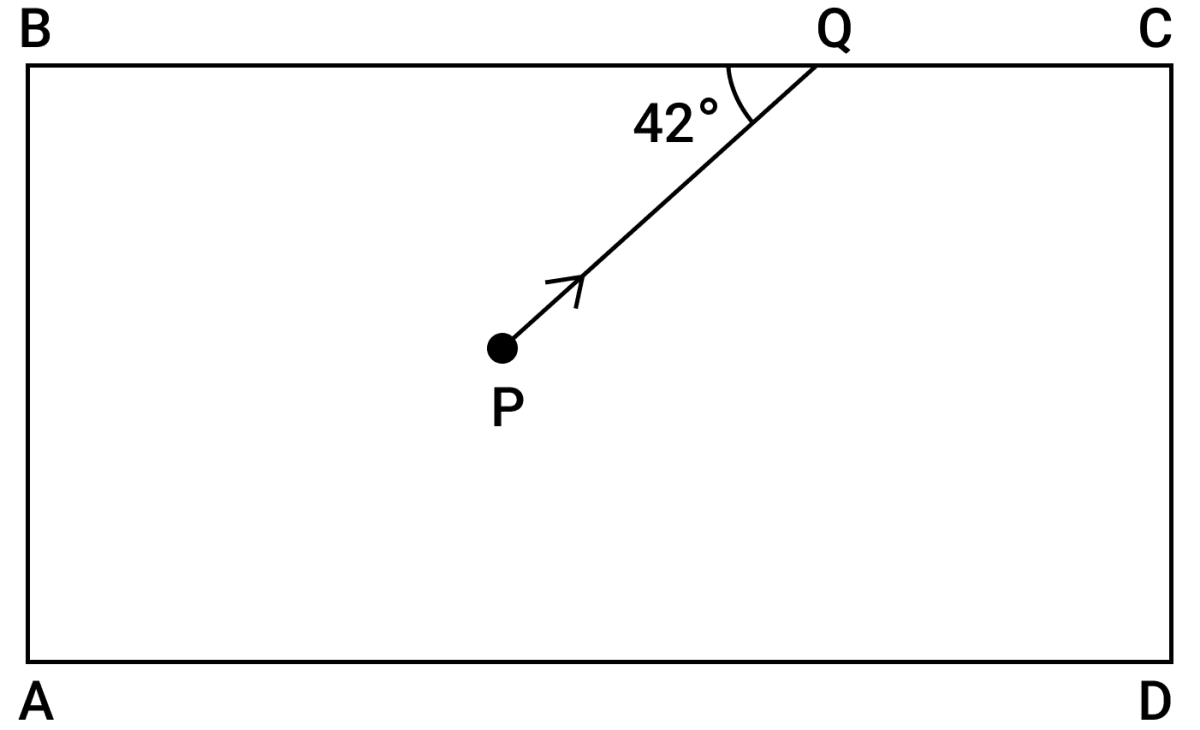The path of ray is as shown: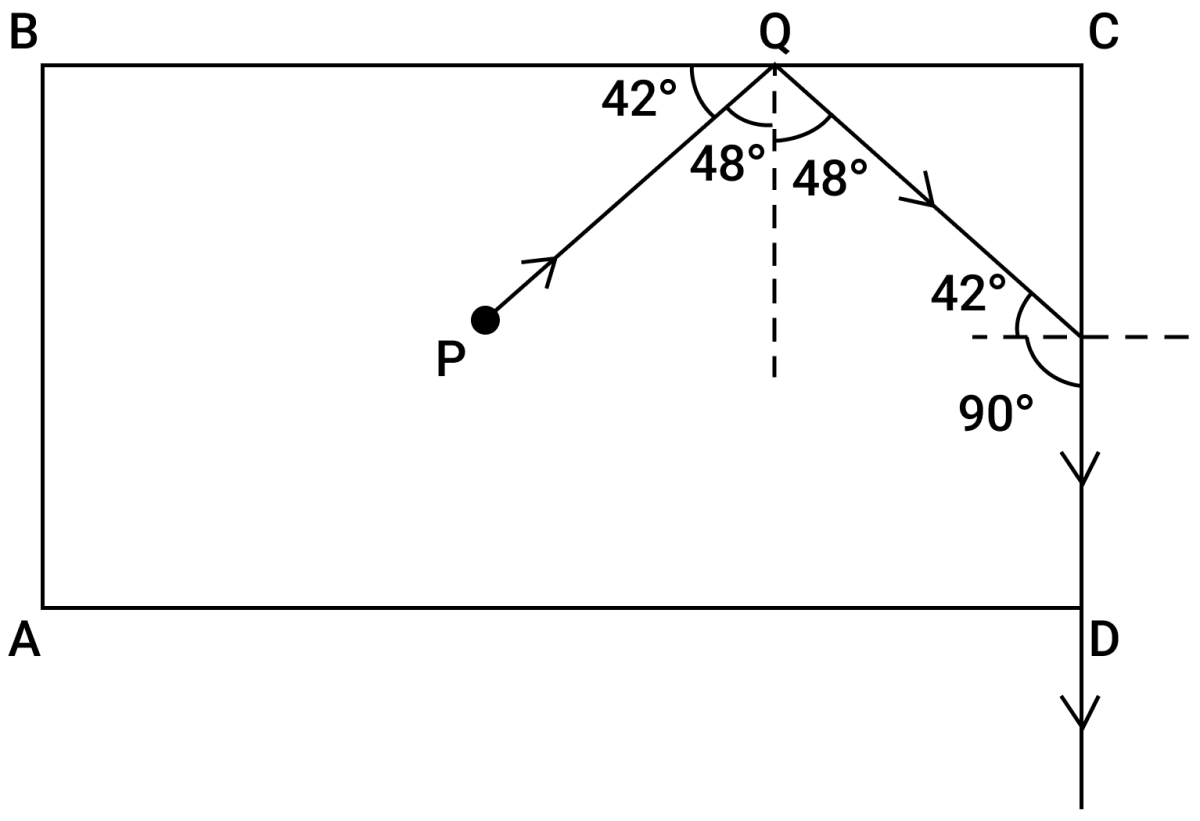#### Question 3(a)

(i) If the lens is placed in water instead of air, how does it's focal length change?

(ii) Which lens, thick or thin has greater focal length? 

(i) Focal length of lens increases.

(ii) A thin lens has greater focal length as compared to a thick lens.

#### Question 3(b)

Two waves of the same pitch have amplitudes in the ratio 1:3. What will be the ratio of their:

(i) intensities and

(ii) frequencies? 

(i) Intensity is the measure of loudness.
Loudness ∝ (amplitude) 2

$\therefore \dfrac{L_1}{L_2} = \Big(\dfrac{a_1}{a_2}\Big)^2 \\[0.5em] \Rightarrow \dfrac{L_1}{L_2} = \Big(\dfrac{1}{3}\Big)^2 \\[0.5em] \Rightarrow \dfrac{L_1}{L_2} = \dfrac{1}{9}$

Therefore, Ratio of intensities is 1:9

(ii) Ratio of frequencies is 1:1 (same frequency as pitch is same for both waves).

#### Question 3(c)

How does an increase in the temperature affect the specific resistance of a :

(i) Metal and

(ii) Semiconductor? 

(i) On increasing the temperature of a metal it's specific resistance increases.

(ii) On increasing the temperature of a semiconductor, it's specific resistance decreases.

#### Question 3(d)

(i) Define resonant vibrations.

(ii) Which characteristic of sound, makes it possible to recognize a person by his voice without seeing him ? 

(i) Resonant vibrations are vibrations of high amplitude which are produced when the natural frequency of vibrations of the vibrating body is equal to the frequency of forced vibration imposed on it.

(ii) Quality (or timbre) is the characteristic of sound that makes it possible to recognize a person by his voice without seeing him.

#### Question 3(e)

Is it possible for a hydrogen ($_1^1\text{H}$) nucleus to emit an alpha particle? Give a reason for your answer. 

No, hydrogen nucleus is just a proton which obviously cannot give out an alpha particle made of two protons and two neutrons.

#### Question 4(a)

Calculate the effective resistance across AB :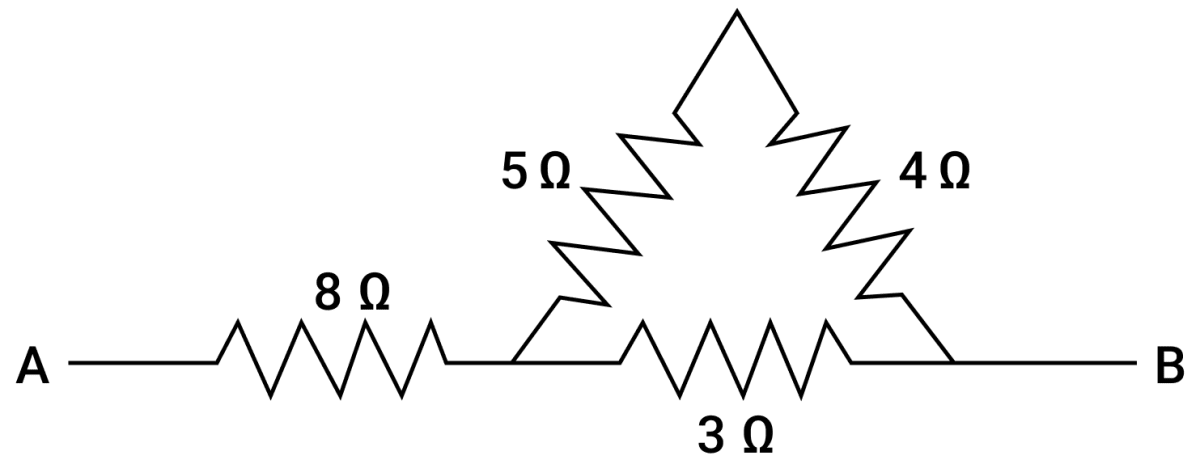The given circuit consists of three parts.

In the first part 5 Ω and 4 Ω are in series and if the equivalent resistance is given by Rs then,

Rs = 5 + 4 = 9 Ω

Then, in second part there is parallel combination of 3 Ω and Rs (9 Ω ), if equivalent resistance is Rp

$\dfrac{1}{R_p} = \dfrac{1}{3} + \dfrac{1}{9} \\[0.5em] \Rightarrow \dfrac{1}{R_p} = \dfrac{3 + 1}{9} \\[0.5em] \Rightarrow \dfrac{1}{R_p} = \dfrac{4}{9} \\[0.5em] \Rightarrow R_p = \dfrac{9}{4} \\[0.5em] \Rightarrow R_p = 2.25 Ω$

In the third part, 8 Ω and Rp ( 2.25 Ω ) are in series. Therefore, the equivalent resistance between AB i.e., RAB is

8 + 2.25 = 10.25 Ω .

Hence, Total resistance between A and B is 10.25 Ω

#### Question 4(b)

(i) State whether the specific heat capacity of a substance remains the same when it's state changes from solid to liquid.

(i) The specific heat capacity of a substance changes when it's state changes.

(ii) The specific heat capacity of water is 4.2 J g-1°C-1 while that of ice (frozen water) is 2.1 J g-1°C-1

#### Question 4(c)

A magnet kept at the centre of two coils A and B is moved to and fro as shown in the diagram. The two galvanometers show deflection.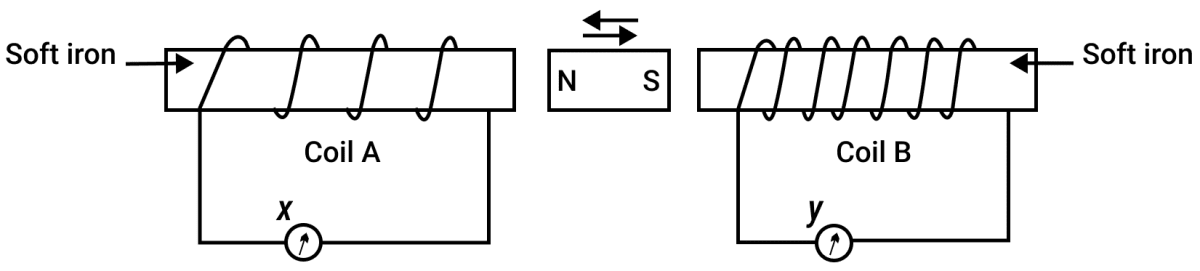State with a reason whether :

x > y

or

x < y [x and y are magnitudes of deflection]

x < y

Since, the coil A has less number of turns, therefore the e.m.f. generated in this coil will be less, hence the galvanometer x connected to this coil shows less deflection i.e., x < y.

#### Question 4(d)

(i) Why is a nuclear fusion reaction called a thermo nuclear reaction? 

(ii) Complete the reaction:

3He2 + 2H14He2 + .......... + Energy

(i) A nuclear fusion reaction is called a thermo nuclear reaction because it takes place at a temperature of nearly 107 K (10 million).

(ii) 3He2 + 2H14He2 + 1H1 + Energy

#### Question 4(e)

State two ways to increase the speed of rotation of a D.C. motor. 

The two ways of increasing the speed of a D.C. motor are

1. By increasing the strength of current through the coil.
2. By increasing the number of turns in the coil.

## Section II (40 Marks)

#### Question 5(a)

A body of mass 10 kg is kept at a height of 5 m. It is allowed to fall and reach the ground. 

(i) What is the total mechanical energy possessed by the body at the height of 2 m assuming it is a frictionless medium?

(ii) What is the kinetic energy possessed by the body just before hitting the ground? Take g = 10 m s-2.

(i) The speed of the body when at a height of 2m above the ground i.e., after falling through 3 m is :

V2 = U2 + 2gh = (0) + (2 x 10 x 3)
= 60

∴ V = √(60) m s-1

Mechanical energy at 2m height = P.E. + K.E. = mgh + $\dfrac{1}{2}$ mv2
= (10 x 10 x 2) + ($\dfrac{1}{2}$ x 10 x 60)
= 200 + 300
= 500 J

Hence, total mechanical energy possessed by the body at the height of 2 m = 500 J

(ii) The kinetic energy on reaching the ground will be same as the potential energy at it's highest point from which it started falling.

Mechanical energy = Initial potential energy

= m x g x h = 10 x 10 x 5 = 500 J

Hence, kinetic energy possessed by the body just before hitting the ground = 500 J

#### Question 5(b)

A uniform metre scale is in equilibrium as shown in the diagram :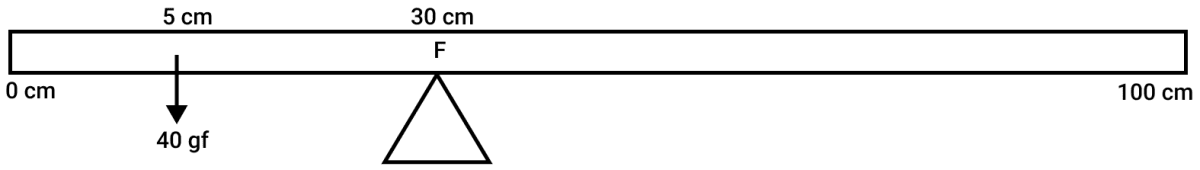(i) Calculate the weight of the metre scale.

(ii) Which of the following options is correct to keep the ruler in equilibrium when 40 gf wt is shifted to 0 cm mark?

F is shifted towards 0 cm

or

F is shifted towards 100 cm.

(i) Let W gf be the weight of metre rule which acts downwards from 50 cm mark.

In equilibrium position, taking moments about fulcrum.

Anticlockwise moment = 40 x (30 - 5) = 1000 gf cm

Clockwise moment = W (50 - 30) = 20 W gf cm

∴ Using principle of moments:
20 W = 1000

⇒ W = $\dfrac{1000}{20}$ = 50 gf

∴ Weight of the metre scale = 50 gf

(ii) In order to keep the ruler in equilibrium, F is shifted towards 0 cm mark.

#### Question 5(c)

The diagram below shows a pulley arrangement :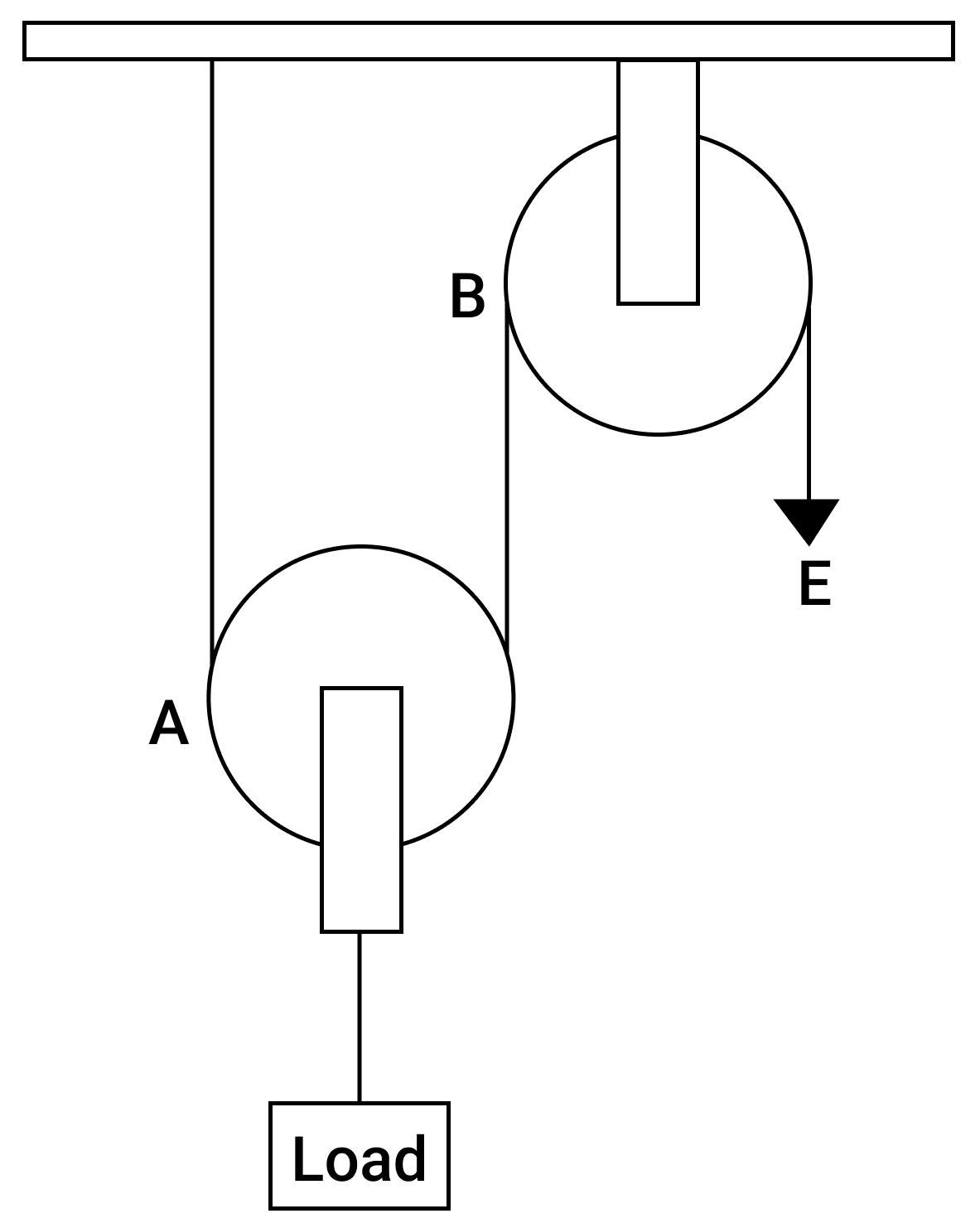(i) Copy the diagram and mark the direction of tension on each strand of the string.

(ii) What is the velocity ratio of the arrangement?

(iii) If the tension acting on the string is T, then what is the relationship between T and effort E?

(iv) If the free end of the string moves through a distance x, find the distance by which the load is raised.

(i) The direction of tension (T) is marked in the diagram: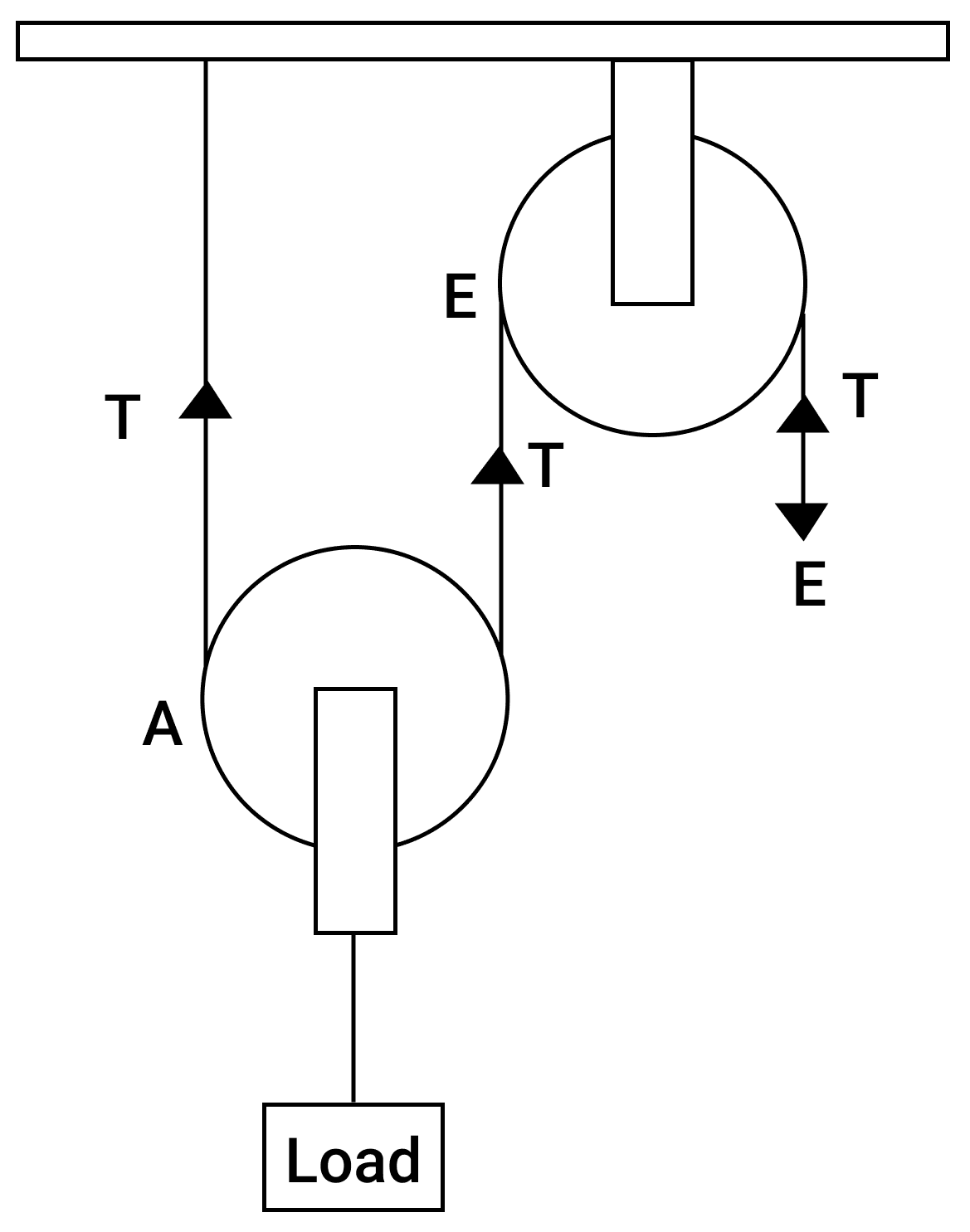(ii) V.R. = $\dfrac{\text{Distance moved by effort}}{\text{Distance moved by load}}$ = 2

(iii) Effort E balances the tensions therefore, T = E

(iv) From the relation,

V.R. = $\dfrac{\text{Distance moved by effort}}{\text{Distance moved by load}}$ = 2

If the free end of the string moves through a distance x then distance moved by load = ?

Substituting the values we get,

$2 = \dfrac{\text{x}}{\text{Distance moved by load}} \\[0.5em] \Rightarrow \text{Distance moved by load} = \dfrac{x}{2}$

#### Question 6(a)

How does the angle of deviation formed by a prism change with the increase in the angle of incidence? Draw a graph showing the variation in the angle of deviation with the angle of incidence at a prism surface. 

The angle of deviation (δ) produced by a prism first decreases with the increase in angle of incidence (i) and reaches to a minimum value at a certain angle of incidence. With further increase in angle of incidence, angle of deviation also increases.

The graph below shows the variation of angle of deviation with the angle of incidence: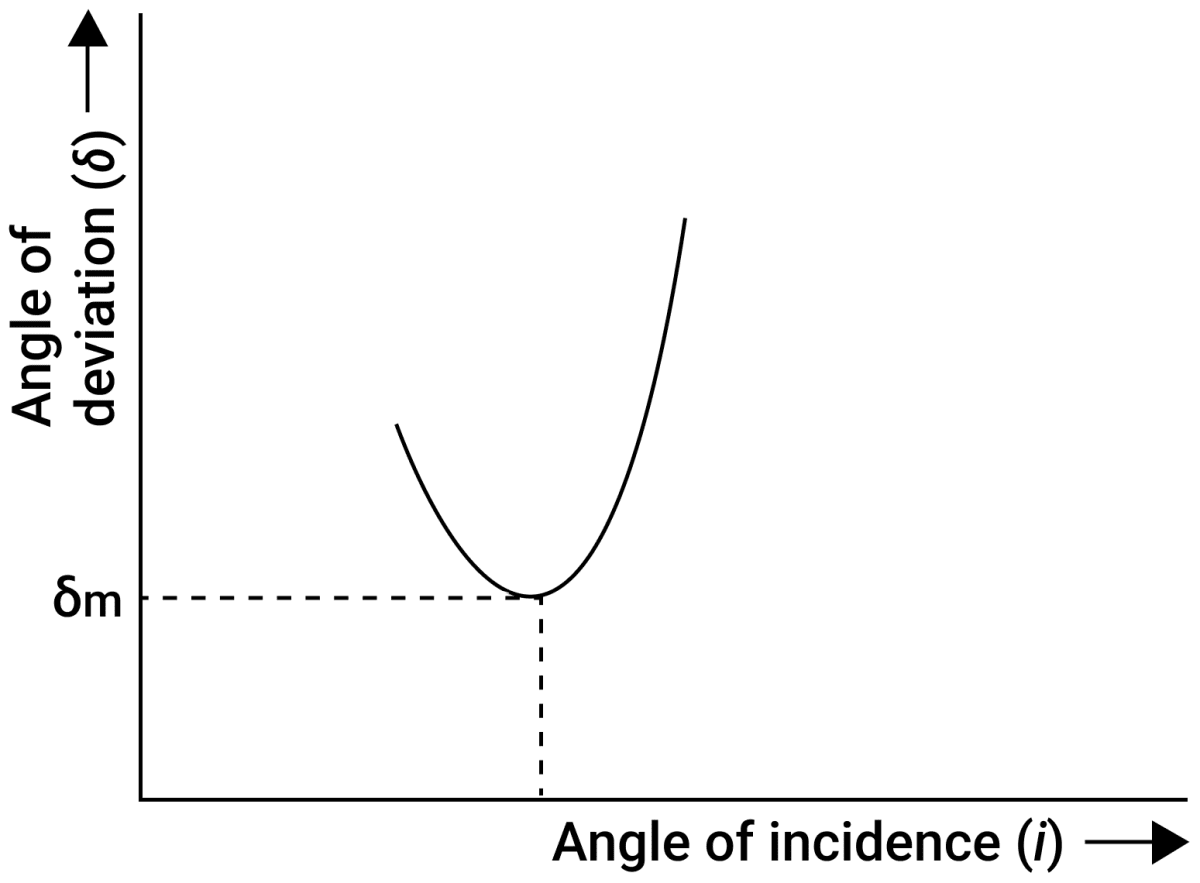#### Question 6(b)

A virtual, diminished image is formed when an object is placed between the optical centre and the principal focus of a lens. 

(i) Name the type of lens which forms the above image.

(ii) Draw a ray diagram to show the formation of the image with the above stated characteristics.

(i) The lens is concave in nature.

(ii) Ray diagram showing the formation of the image is given below: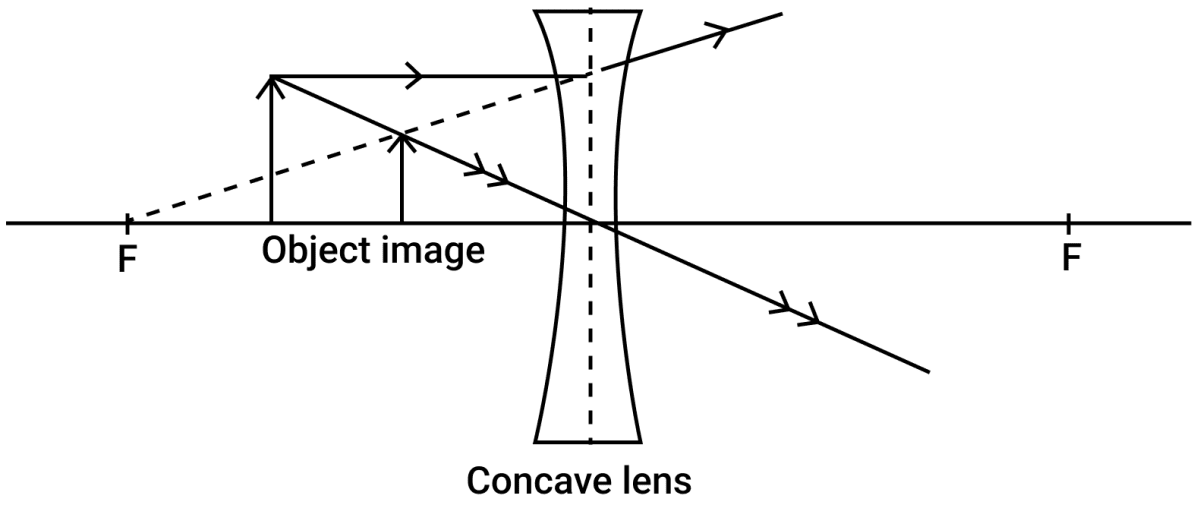#### Question 6(c)

An object is placed at a distance 24 cm in front of a convex lens of focal length 8 cm. 

(i) What is the nature of the image so formed ?

(ii) Calculate the distance of the image from the lens.

(iii) Calculate the magnification of the image.

Given u = 24 cm (-ve), f = 8 cm (+ve)

(i) Image formed is real, inverted and diminished.

(ii) Let v be the distance of image from optical centre. From the relation,

$\dfrac{1}{v} - \dfrac{1}{u} = \dfrac{1}{f} \\[0.5em]$

Substituting the values we get,

$\dfrac{1}{v} + \dfrac{1}{24} = \dfrac{1}{8} \\[0.5em] \dfrac{1}{v} = \dfrac{1}{8} - \dfrac{1}{24} \\[0.5em] \dfrac{1}{v} = \dfrac{3 - 1}{24} \\[0.5em] \dfrac{1}{v} = \dfrac{2}{24} \\[0.5em] \dfrac{1}{v} = \dfrac{1}{12} \\[0.5em] \Rightarrow v = 12 \text{cm}$

Hence, the distance of the image from the lens = 12 cm

(iii) From relation, m = $\dfrac{v}{u}$

Substituting the values we get,

$m = \dfrac{12}{24} \\[0.5em] \Rightarrow m = 0.5$

Hence, magnification = 0.5

#### Question 7(a)

It is observed that during march-past we hear a base drum distinctly from a distance compared to the side drums. 

(i) Name the characteristic of sound associated with the above observation.

(ii) Give a reason for the above observation.

(i) Loudness

(ii) Base drum has a greater surface area compared to the side drums so amplitude of vibration of base drum is greater than amplitude of vibration of side drums. Hence Loudness of base drum is increased compared to the side drums and it can be distinctly heard from a distance.

#### Question 7(b)

A pendulum has a frequency of 4 vibrations per second. An observer starts the pendulum and fires a gun simultaneously. He hears the echo from the cliff after 6 vibrations of the pendulum. If the velocity of sound in air is 340 m s-1, find the distance between the cliff and the observer. 

Echo is heard after 6 vibrations of pendulum. Since frequency of pendulum is 4 vib per sec, so the time taken for the echo to be heard is t = $\dfrac{6}{4}$ = 1.5 s.

If d is the distance between the cliff and the observer, then velocity of sound V = $\dfrac{2d}{t}$

Substituting the values in the equation above:

$340 = \dfrac{2d}{1.5} \\[0.5em] \Rightarrow d = \dfrac{340 \times 1.5}{2} = 255 \text{ cm}$

Hence, distance between the cliff and the observer = 255 cm

#### Question 7(c)

Two pendulums C and D are suspended from a wire as shown in the given figure. Pendulum C is made to oscillate by displacing it from it's mean position. It is seen that D also starts oscillating.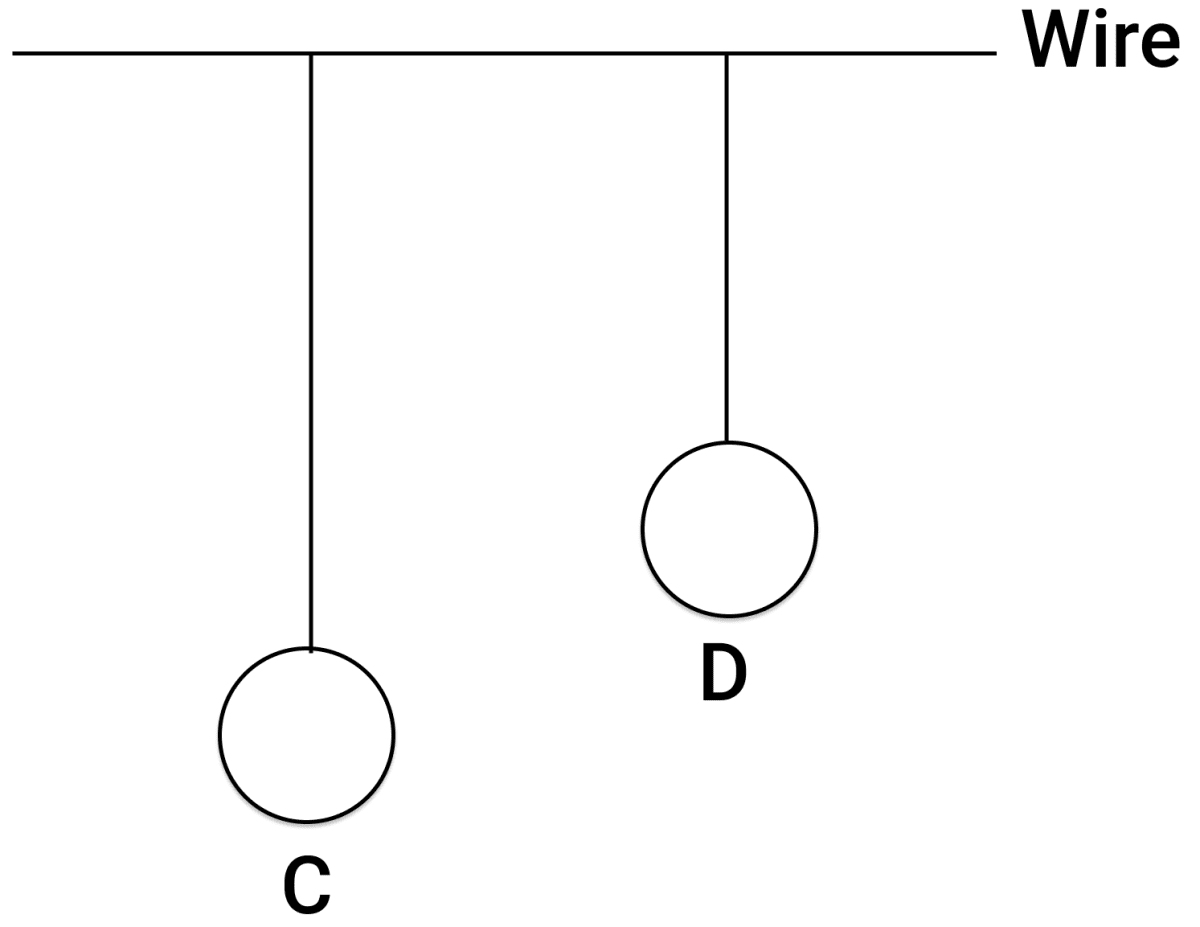(i) Name the type of oscillation, C will execute.

(ii) Name the type of oscillation, D will execute.

(iii) If the length of D is made equal to C, then what difference will you notice in the oscillations of D?

(iv) What is the name of the phenomenon when the length of D is made equal to C?

(i) C executes free vibrations (damped if medium is present).

(ii) D executes forced oscillation.

(iii) If the length of D is made equal to C, then the amplitude of vibration of pendulum D increases substantially.

(iv) The phenomenon is called resonance.

#### Question 8(a)

(i) Write one advantage of connecting electrical appliances in parallel combination.

(ii) What characteristics should a fuse wire have?

(iii) Which wire in a power circuit is connected to the metallic body of the appliance? 

(i) The advantage of connecting electrical appliances in parallel combination is that each appliance works independently i.e., any change in one appliance does not affect the other appliance(s).

(ii) The fuse wire should have high specific resistance and low melting point.

(iii) The earth wire in a power circuit is connected to the metallic body of the appliance

#### Question 8(b)

The diagram below shows a dual control switch circuit connected to a bulb.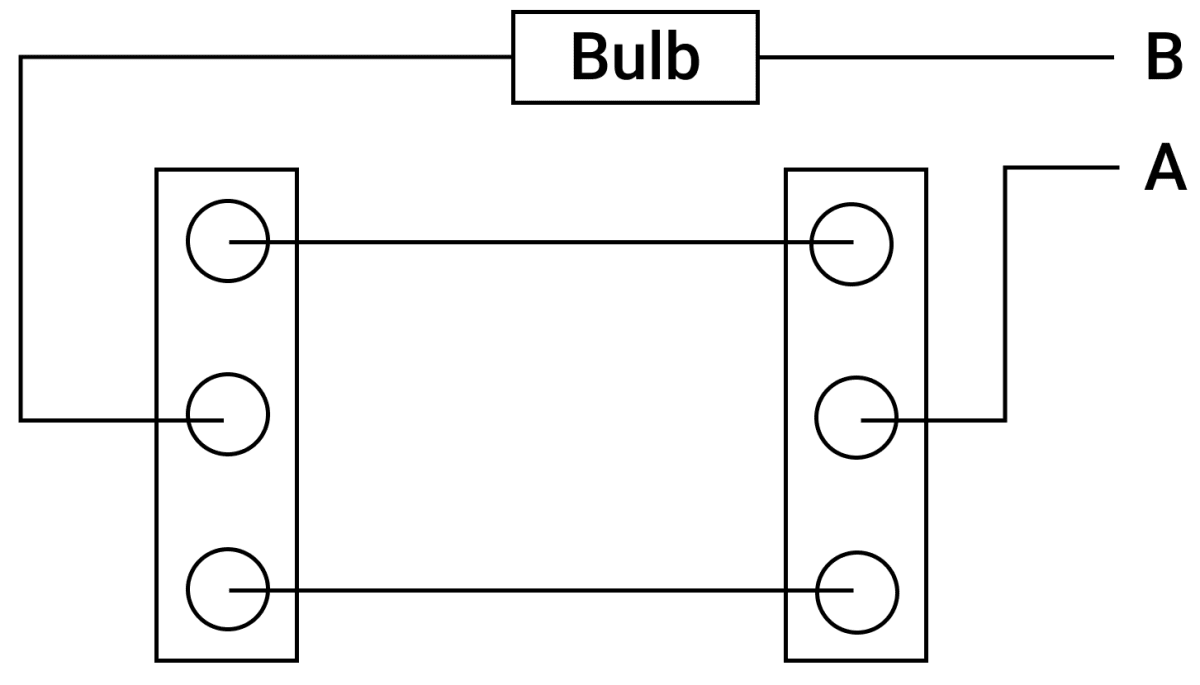(i) Copy the diagram and complete it so that the bulb is switched ON.

(ii) Out of A & B which one is the live wire and which one is the neutral wire?

(i) The following figure shows the completed diagram: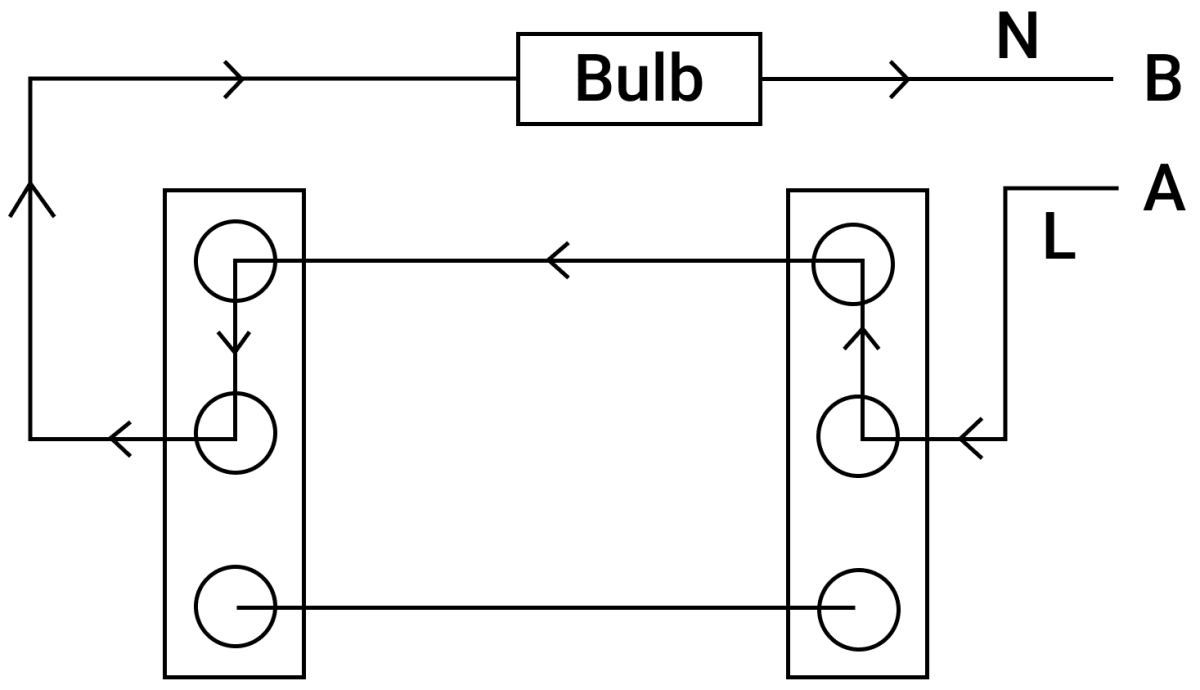(ii) Wire A is the live wire and wire B is the neutral wire.

#### Question 8(c)

The diagram shows a circuit with the key k open. Calculate: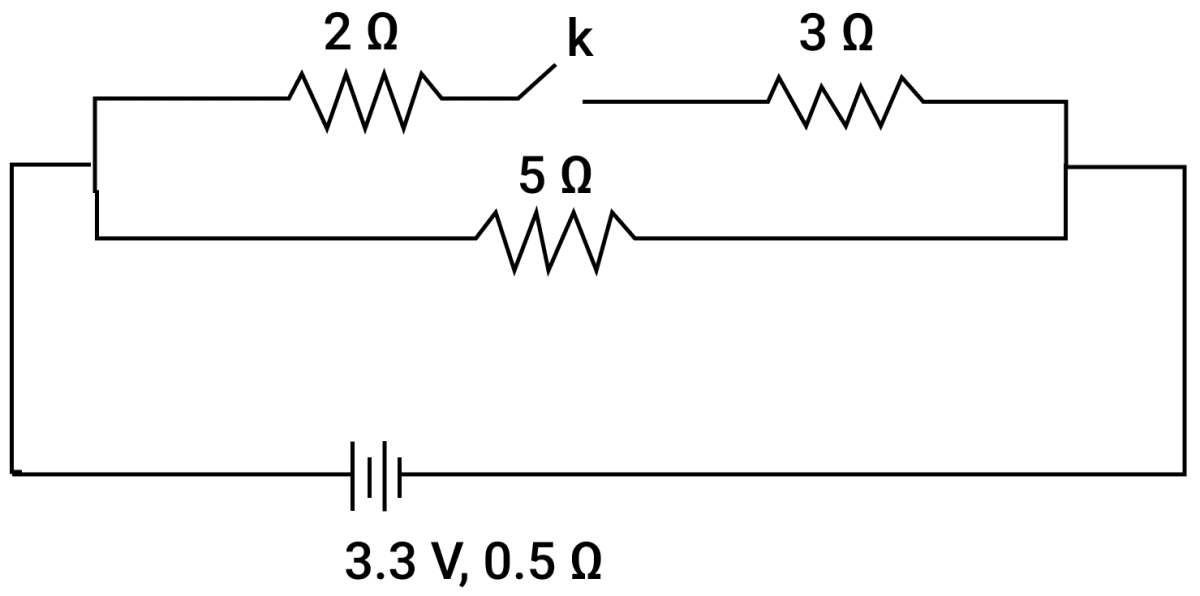(i) the resistance of the circuit when the key k is open.

(ii) the current drawn from the cell when the key k is open.

(iii) the resistance of the circuit when the key k is closed.

(iv) the current drawn from the cell when the key k is closed.

(i) When k is open, resistance is 5 + 0.5 = 5.5 Ω

(ii) From relation, V = IR

Substituting the values in the formula we get,

$3.3 = \text{I} \times 5.5 \\[0.5em] \Rightarrow \text{I} = \dfrac{3.3}{5.5} \\[0.5em] \Rightarrow \text{I} = 0.6 \text{ A}$

Hence, current drawn from the cell when the key k is open = 0.6 A.

(iii) When k is closed, the resistance 2 Ω and 3 Ω are in series, therefore their total resistance is 2 Ω + 3 Ω = 5 Ω . Now since, 5 Ω resistance is in parallel to it,

Therefore,

$\dfrac{1}{R_p} = \dfrac{1}{5} + \dfrac{1}{5} \\[0.5em] \dfrac{1}{R_p} = \dfrac{1 + 1}{5} \\[0.5em] \Rightarrow \dfrac{1}{R_p} = \dfrac{2}{5} \\[0.5em] \Rightarrow R_p = 2.5 Ω$

So now the resistance of circuit is 2.5 + 0.5 = 3 Ω

(iv) Current drawn I = $\dfrac{\text{V}}{\text{R}}$

Substituting the values we get,

$\text{I} = \dfrac{3.3}{3} \\[0.5em] \Rightarrow \text{I} = 1.1 \text{ A}$

#### Question 9(a)

(i) Define Calorimetry. 

(ii) Name the material used for making a Calorimeter.

(iii) Why is a Calorimeter made-up of thin sheets of the above material answered in (ii) ?

(i) The science of measuring the amount of heat exchanged by the system with it's surrounding is called calorimetry.

(ii) A copper sheet is used to make a calorimeter.

(iii) It is because Copper is a good conductor of heat so it soon acquires the temperature of it's contents and a thin Copper sheet has low heat capacity, so it takes only a small amount of heat from it's contents. Hence, a Calorimeter is made-up of thin sheets of Copper.

#### Question 9(b)

The melting point of naphthalene is 80°C and the room temperature is 30°C. A sample of liquid naphthalene at 100°C is cooled down to the room temperature. Draw a temperature time graph to represent this cooling. In the graph, mark the region which corresponds to the freezing process. 

The temperature-time graph representing the cooling of naphthalene is shown below: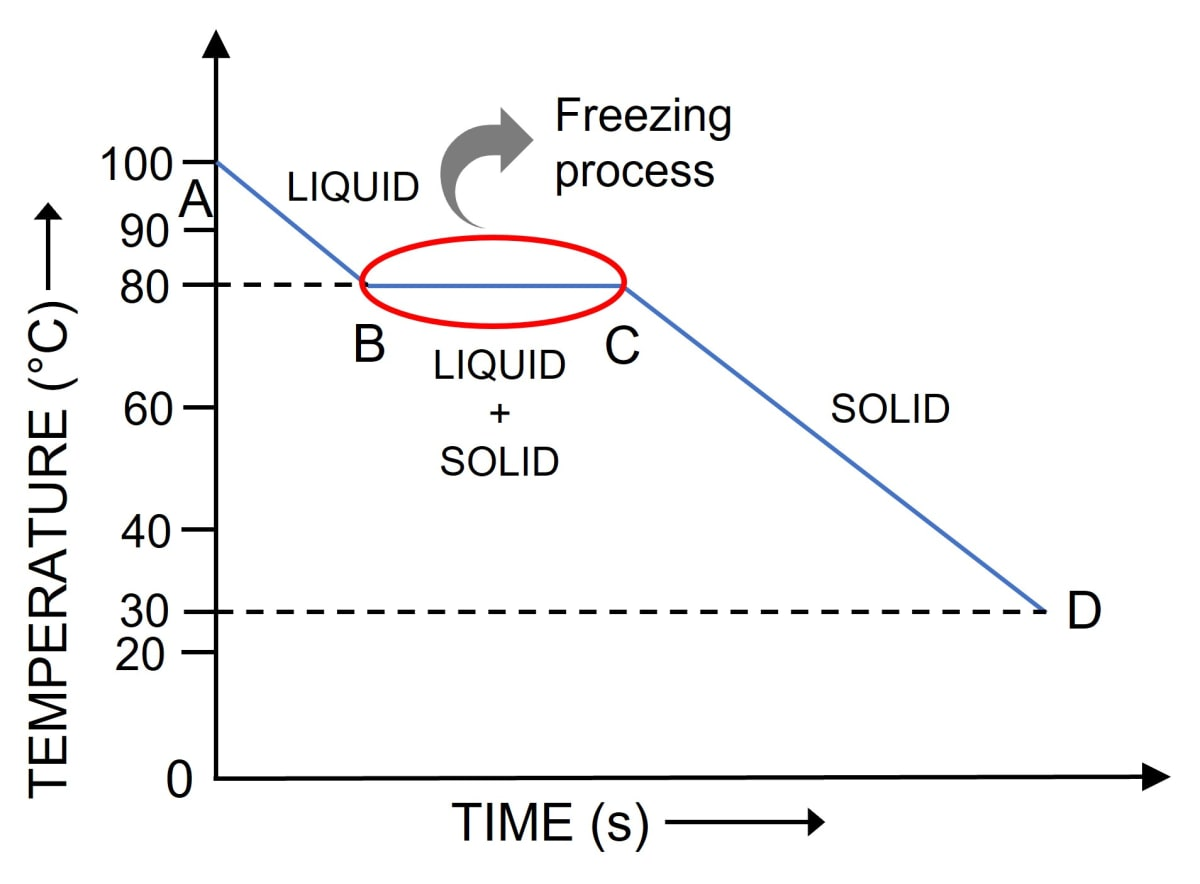#### Question 9(c)

104 g of water at 30°C is taken in a calorimeter made of copper of mass 42 g. When a certain mass of ice at 0°C is added to it, the final steady temperature of the mixture after the ice has melted, was found to be 10°C. Find the mass of ice added.
[Specific heat capacity of water = 4.2 J g-1 °C-1; Specific latent heat of fusion of ice = 336 J g-1; Specific heat capacity of copper = 0.4 J g-1 °C-1 ] 

Given,

Mass of water = 104 g

Temperature of water = 30°C

Final temperature = 10°C

Mass of calorimeter = 42 g

Temperature of ice = 0°C

The heat given by water = m x s x t

Substituting the values we get,

The heat given by water = 104 x 4.2 x (30 - 10) = 8736 J

The heat given by calorimeter = m x s x t

Substituting the values we get,

The heat given by calorimeter = 42 x 0.4 x (30 - 10) = 336 J

Let mass of ice added be m gm

Heat taken by ice to melt = mL = 336m J

Heat taken by ice-water to reach the mixture temperature = mst = m x 4.2 x (10 - 0) = 42m J

Therefore, from principle of calorimetry, heat taken = heat given.

i.e., 336 m + 42 m = 8736 + 336

Therefore, m = $\dfrac{9072}{378}$ = 24 g

Hence, mass of ice added = 24 g

#### Question 10(a)

Draw a neat labelled diagram of an AC generator. 

Labelled diagram of an AC generator is as shown: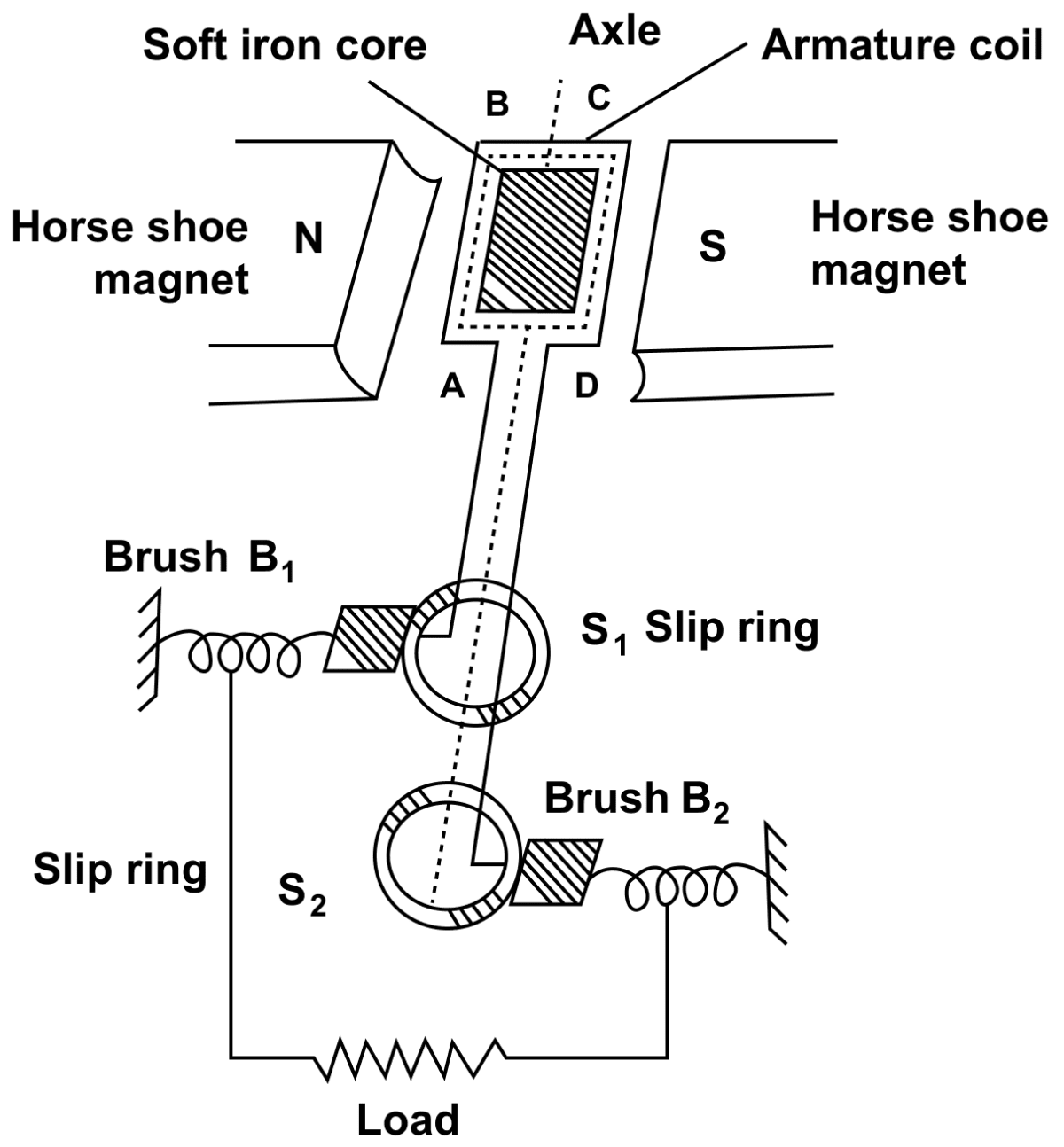#### Question 10(b)

(i) Define nuclear fission. 

(ii) Rewrite and complete the following nuclear reaction by filling in the atomic number of Ba and mass number of Kr :

$_{92}^{235}\text{U}$ + $_{0}^{1}\text{n}$$_{...}^{144}\text{Ba}$ + $_{36}^{...}\text{Kr}$ + 3 $_{0}^{1}\text{n}$ + Energy

(i) The process of splitting of a heavy nucleus into two lighter nuclei of nearly same mass, when bombarded with slow neutrons, is called nuclear fission.

(ii) $_{92}^{235}\text{U}$ + $_{0}^{1}\text{n}$$_{56}^{144}\text{Ba}$ + $_{36}^{89}\text{Kr}$ + 3 $_{0}^{1}\text{n}$ + Energy

#### Question 10(c)

The diagram below shows a magnetic needle kept just below the conductor AB which is kept in North South direction.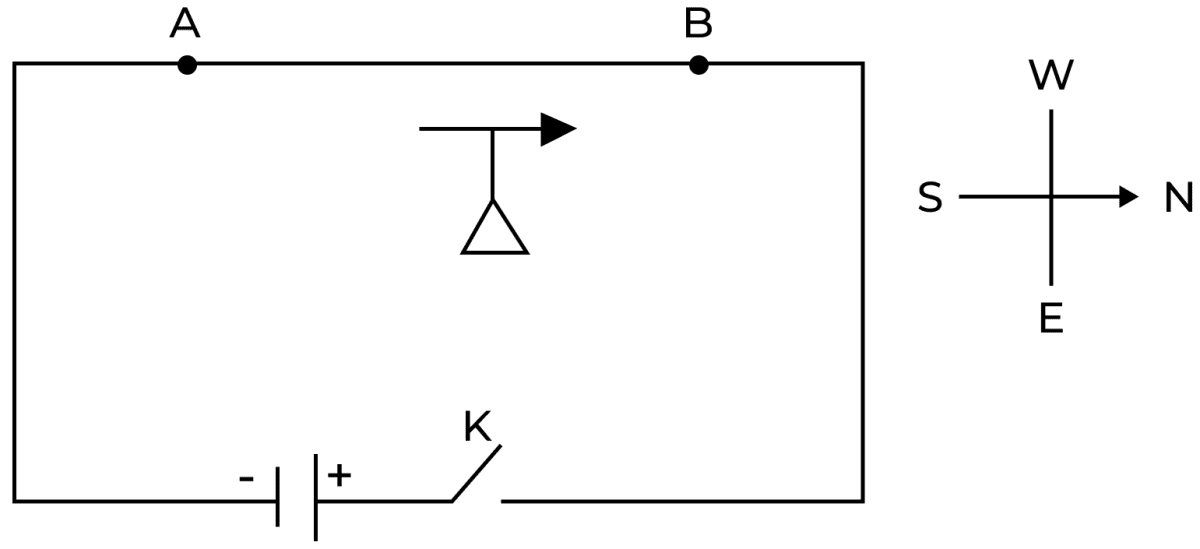(i) In which direction does the needle deflect when the key is closed ?

(ii) Why is the deflection produced?

(iii) What will be the change in the deflection if the magnetic needle is taken just above the conductor AB ?

(iv) Name one device which works on this principle.